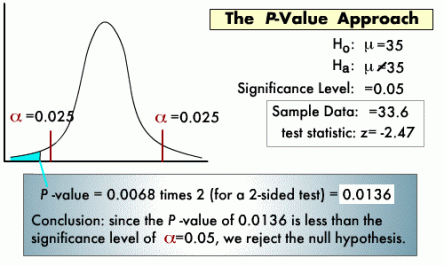P – The \$ixteenth Letter Of The English AlphabetP, p

[pee]

noun, plural P’s or Ps, p’s or ps.
1. the sixteenth letter of the English alphabet, a consonant.

2. any spoken sound represented by the letter P or p, as in pet, supper, top, etc.

3. something having the shape of a P .

4. a written or printed representation of the letter P or p.

5. a device, as a printer’s type, for reproducing the letter P or p.P Value (Economics)

Definition: P value is associated with a test statistic. It is “the probability, if the test statistic really were distributed as it would be under the null hypothesis, of observing a test statistic [as extreme as, or more extreme than] the one actually observed.”

The smaller the P value, the more strongly the test rejects the null hypothesis, that is, the hypothesis being tested.

A p-value of .05 or less rejects the null hypothesis “at the 5% level” that is, the statistical assumptions used imply that only 5% of the time would the supposed statistical process produce a finding this extreme if the null hypothesis were true.

5% and 10% are common significance levels to which p-values are compared.

A different (p)erspective to consider.